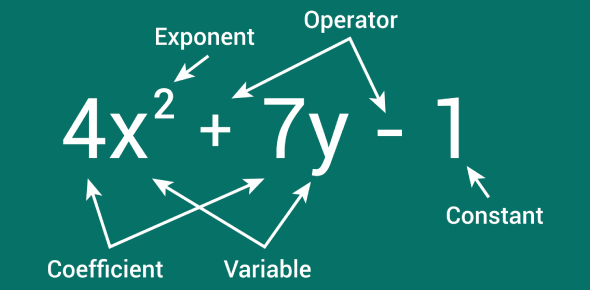# Simplify A Polynomial Expression Quiz

Approved & Edited by ProProfs Editorial Team
The editorial team at ProProfs Quizzes consists of a select group of subject experts, trivia writers, and quiz masters who have authored over 10,000 quizzes taken by more than 100 million users. This team includes our in-house seasoned quiz moderators and subject matter experts. Our editorial experts, spread across the world, are rigorously trained using our comprehensive guidelines to ensure that you receive the highest quality quizzes.
| By Jamessteve
J
Jamessteve
Community Contributor
Quizzes Created: 234 | Total Attempts: 1,299,688
Questions: 10 | Attempts: 932Settings• 1.

### 7x-11+7x+11

• A.

11x

• B.

14x

• C.

5x

• D.

8x

B. 14x
Explanation
The given expression is 7x - 11 + 7x + 11. By combining like terms, we can simplify it to 14x. The coefficients of the x terms (7x and 7x) add up to 14x, while the constants (-11 and 11) cancel each other out. Therefore, the simplified expression is 14x.

Rate this question:

• 2.

### 5x+2-3x

• A.

2x+2

• B.

2

• C.

-2

• D.

1

A. 2x+2
Explanation
The given expression is 5x+2-3x. To simplify this expression, we combine like terms. The x terms, 5x and -3x, can be added together to give 2x. The constant terms, 2 and 0, can be added together to give 2. Therefore, the simplified expression is 2x+2.

Rate this question:

• 3.

### -3y-3+6x+3y-10

• A.

16x-13

• B.

10x-3

• C.

2x+10

• D.

None

D. None
Explanation
The given expression -3y-3+6x+3y-10 simplifies to 6x-13. None of the given answer choices match this simplified expression. Therefore, the correct answer is "None".

Rate this question:

• 4.

### 4x-x

• A.

None

• B.

3x

• C.

2

• D.

X

B. 3x
Explanation
The expression 4x - x can be simplified by subtracting x from 4x, which gives us 3x. Therefore, the correct answer is 3x.

Rate this question:

• 5.

### 7a + 8 - 3a

• A.

4a+8

• B.

15a+3a

• C.

7a+8

• D.

8a+5a

A. 4a+8
Explanation
The expression 7a + 8 - 3a simplifies to 4a + 8. This is because when we combine like terms, we add the coefficients of the same variable. Here, the coefficients of 'a' are 7 and -3. So, when we subtract 3a from 7a, we get 4a. The constant term 8 remains unchanged. Therefore, the simplified expression is 4a + 8.

Rate this question:

• 6.

### 3x +4x?

• A.

10x

• B.

7x

• C.

8x

• D.

None

B. 7x
Explanation
The given expression is 3x + 4x. To simplify this expression, we can combine the like terms, which in this case are the terms with the variable x. Adding 3x and 4x gives us a total of 7x. Therefore, the correct answer is 7x.

Rate this question:

• 7.

### Combine the like terms. 8y + 5y

• A.

8y

• B.

10y

• C.

13y

• D.

15y

C. 13y
Explanation
When combining like terms, we add the coefficients of the terms with the same variable. In this case, we have 8y and 5y. Adding the coefficients 8 and 5 gives us 13. Therefore, the answer is 13y.

Rate this question:

• 8.

### 16-3x+2-5y+10x

• A.

7x-5y+18

• B.

2x+2

• C.

17x+3

• D.

None

A. 7x-5y+18
Explanation
The given expression can be simplified by combining like terms. We can combine the terms with 'x' and the terms with 'y'. Combining the terms with 'x', we get -3x + 10x = 7x. Combining the terms with 'y', we get -5y. The constant terms 16 and 2 can be combined to give 18. Therefore, the simplified expression is 7x - 5y + 18.

Rate this question:

• 9.

### -20x+x-12+3y-6-7x

• A.

15

• B.

12x+y

• C.

-25x+2y-18

• D.

-26x+3y-18

A. 15
Explanation
The given expression is simplified by combining like terms. By combining the terms -20x and -7x, we get -27x. Similarly, combining x and 3y, we get 4y. Combining -12, -6, and 15, we get -3. Therefore, the simplified expression is -27x + 4y - 3. However, this does not match any of the given answer choices. Therefore, the correct answer is not provided.

Rate this question:

• 10.

### 15y+27-15y-20-7

• A.

7x-11

• B.

1

• C.

0

• D.

12

C. 0
Explanation
The given expression is 15y + 27 - 15y - 20 - 7. Simplifying this expression, we can combine like terms. The 15y and -15y cancel each other out, leaving us with 27 - 20 - 7. Simplifying further, we get 7 - 7, which equals 0. Therefore, the correct answer is 0.

Rate this question:

Related TopicsBack to top